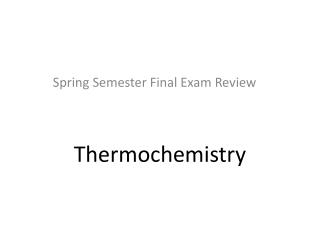DownloadDownload PresentationThermochemistry

# Thermochemistry

Download Presentation## Thermochemistry

- - - - - - - - - - - - - - - - - - - - - - - - - - - E N D - - - - - - - - - - - - - - - - - - - - - - - - - - -
##### Presentation Transcript

1. Thermochemistry Spring Semester Final Exam Review

2. Fuel is burnt to produce energy - combustion (e.g. when fossil fuels are burnt) CH4(g) + 2O2(g) CO2(g) + 2H2O(l) + energy THERMOCHEMISTRY The study of heat released or required by chemical reactions

3. Energy Kinetic energy (KE) Potential energy (PE) Energy due to motion Stored energy What is Energy?

4. Total Energy = Kinetic Energy + Potential Energy E = KE + PE Temperature measures the average Kinetic energy & potential energy are interchangeable Heat is the total energy of a system: Kinetic energy + potential energy

5. Systems & Surroundings In thermodynamics, the world is divided into a system and its surroundings A systemis the part of the world we want to study (e.g. a reaction mixture in a flask) The surroundingsconsist of everything else outside the system

6. Burning fossil fuels is an exothermic reaction EXOTHERMIC & ENDOTHERMIC REACTIONS Exothermic process: a change (e.g. a chemical reaction) that releases heat to the surroundings. A release of heat corresponds to a decrease in enthalpy Exothermic process: H < 0 (at constant pressure)

7. Exothermic Reactions

8. Endothermic process: a change (e.g. a chemical reaction) that requires (or absorbs) heat from the surroundings. An input of heat corresponds to an increase in enthalpy Endothermic process: H > 0 (at constant pressure) Photosynthesis is an endothermic reaction (requires energy input from sun)

9. Endothermic Reactions

10. Endothermic or Exothermic? exothermic endothermic exothermic endothermic endothermic

11. Heating Curves Heating Curves Animation A plot of temperature vs. time that represents the process in which energy is added at a constant rate Courtesy Christy Johannesson www.nisd.net/communicationsarts/pages/chem

12. Gas - KE  Boiling - PE  Liquid - KE  Melting - PE  Solid - KE  Heating Curves 140 120 100 80 60 40 Temperature (oC) 20 0 -20 -40 -60 -80 -100 Time

13. A plot of temperature vs. time that represents the process in which energy is added at a constant rate

14. The standard enthalpy of reaction(DH0 ) is the enthalpy of a reaction carried out at 1 atm. rxn aA + bBcC + dD - [ + ] [ + ] = - S S = DH0 DH0 rxn rxn mDH0 (reactants) dDH0 (D) nDH0 (products) cDH0 (C) bDH0 (B) aDH0 (A) f f f f f f 6.6

15. Example Problem Calculate the heat of combustion of methane, CH4 CH4(g) +2O2(g) CO2(g) + 2 H2O(g) H◦fCH4 (g) = -74.86 kJ/mol H◦fO2(g) = 0 kJ/mol H◦fCO2(g) = -393.5 kJ/mol H◦fH2O(g) = -241.8 kJ/mol pg. 316 2 mol(-241.8 kJ/mol) =-483.6 kJ Step #1: multiply the H◦fH2O(g) by 2 since there are two moles of water in the products .

16. Example Problem Calculate the heat of combustion of methane, CH4 CH4(g) +2O2(g) CO2(g) + 2 H2O(g) H◦fCH4 (g) = -74.86 kJ H◦fO2(g) = 0 kJ/ H◦fCO2(g) = -393.5 kJ H◦ fH2O(g) = -483.6 kJ pg. 316 H◦f=[-393.5 kJ + (-483.6 kJ)]- [-74.86 kJ + (0 kJ )] H◦f= -802.2 kJ Step #2: sum up all the H◦f. : Hrxn = Hf(products) - Hf(reactants)

17. Calculations Involving Specific Heat OR C= Specific Heat Capacity q = Heat lost or gained T = Temperature change Tf - Ti

18. Choose all that apply... C(s) + 2 S(g) CS2(l) H = 89.3 kJ Which of the following are true? • This reaction is exothermic B) It could also be written C(s) + 2 S(g) + 89.3 kJ CS2(l) C) The products have higher energy than the reactants D) It would make the water in the calorimeter colder